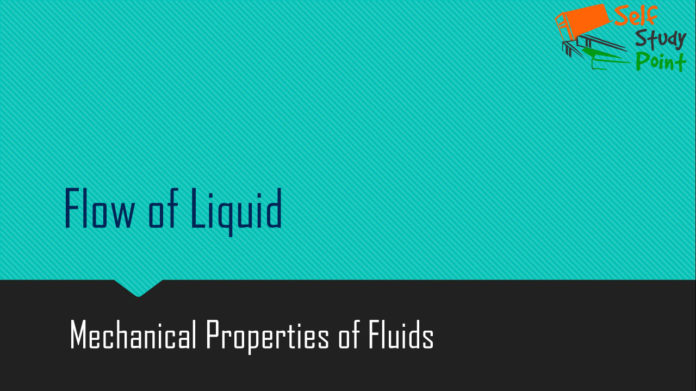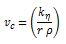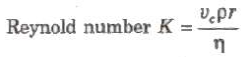## Streamline Flow

The flow of liquid in which each of its particle follows the same path as followed by the proceeding particles, is called streamline flow.

## Laminar Flow

The steady flow of liquid over a horizontal surface in the form of layers of different velocities is called laminar flow.

## Turbulent Flow

The flow of liquid with a velocity greater than its critical velocity is disordered and called turbulent flow.

## Critical Velocity

The critical velocity is that velocity of liquid flow, below which its fl is streamlined and above which it becomes turbulent.

Critical velocity,Where,

K = Reynolds’s number,

η = coefficient of viscosity of liquid

r = radius of capillary tube and

ρ = density of the liquid.

## Reynolds’s Number

Reynolds’s number is a pure number and it is equal to the ratio of inertial force per unit area to the viscous force per unit area for flowing fluid.Where p = density of the liquid and vc = critical velocity. For pure water flowing in a cylindrical pipe, K is about 1000.

When 0< K< 2000, the flow of liquid is streamlined.

When 2000 < K < 3000, the flow of liquid is variable between streamlined and turbulent.

When K > 3000, the flow of liquid is turbulent.

It has no unit and dimension.

#### 1 COMMENT

1.sooraj rawat

You have written a very good article, I have fully understood, you have cleared all the doubts, thank you very much for this article.